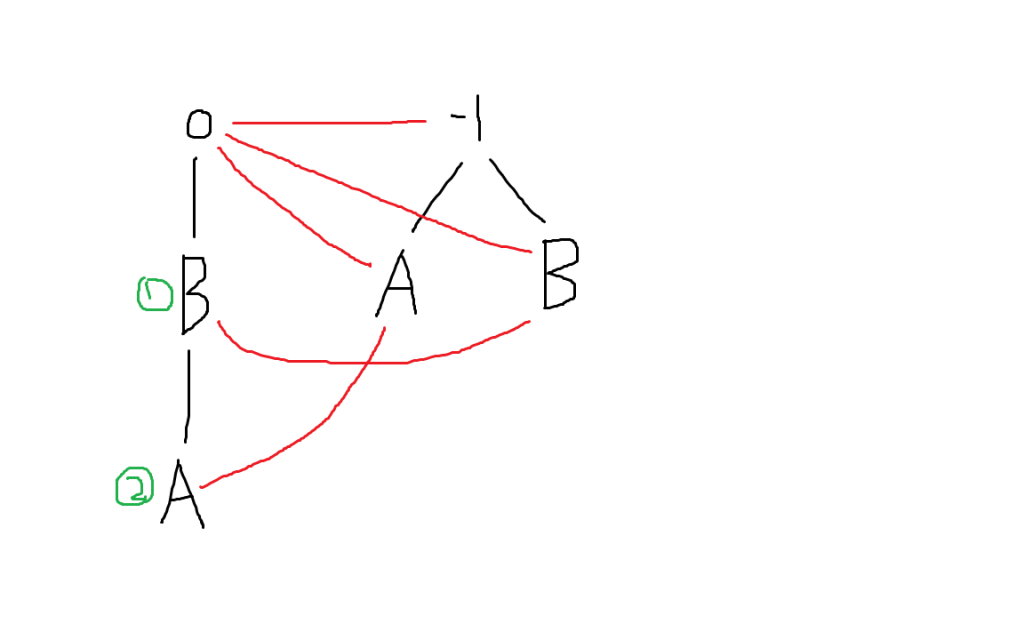# 回文自动机

written by oneman233
2019-09-131. 上图中黑线表示后缀边，红线表示$fail$边
2. 每个节点都代表了一个回文子串，例如图中①号节点代表了$BB$，②号节点代表了$ABBA$
3. 一个回文自动机实际上包含两棵树，分别代表奇偶回文子串
4. 每条后缀边上都有一个字符，若某点对应的回文子串为$S$，后缀边上的字符为$c$，那么这条后缀边指向了代表$c+S+c$这个回文串的节点
5. 把奇数回文子串的初始长度设为$-1$就是为了满足后缀边的特性

/*
fail[x]：x节点失配之后跳转到不等于自身的最长后缀回文子串
len[x]：以x结尾的最长回文子串长度
num[x]： 与”以x结尾的最长回文子串“本质不同的子串个数
cnt[x]：与”以x结尾的最长回文子串“本质相同的子串个数
（注意上面两个数组完全相反）
son[x][c]：编号为x的节点表示的回文子串在两边添加字符c之后变成的回文子串编号
s[x]：第x次添加的字符，s数组即原字符串
tot：总节点个数，节点编号由0到tot-1
last：最后一个新建立节点的编号
cur：当前节点在PAM上的父亲编号
*/
struct PAM
{
int tot,last,n,cur;
int fail[N],len[N],same[N],diff[N],son[N];
char s[N];
int get(int p,int x)
{
while(s[x-len[p]-1]!=s[x])
p=fail[p];
return p;
}
int newnode(int x)
{
len[tot]=x;
}
void build()
{
scanf("%s",s+1);
s=-1,fail=1,last=0;
newnode(0),newnode(-1);
for(n=1;s[n];++n)
{
s[n]-='a';
cur=get(last,n);
if(!son[cur][s[n]])
{
int now=newnode(len[cur]+2);
fail[now]=son[get(fail[cur],n)][s[n]];
diff[now]=diff[fail[diff[now]]]+1;
son[cur][s[n]]=now;
}
same[last=son[cur][s[n]]]++;
}
for(int i=tot-1;i>=0;--i)
same[fail[i]]+=same[i];
}
}pam;